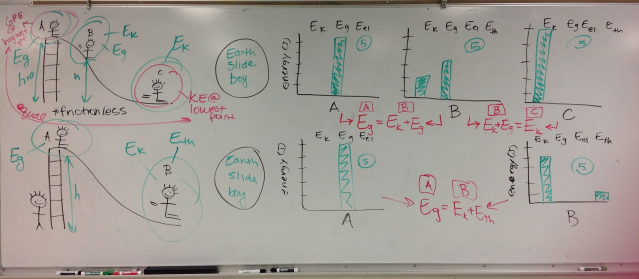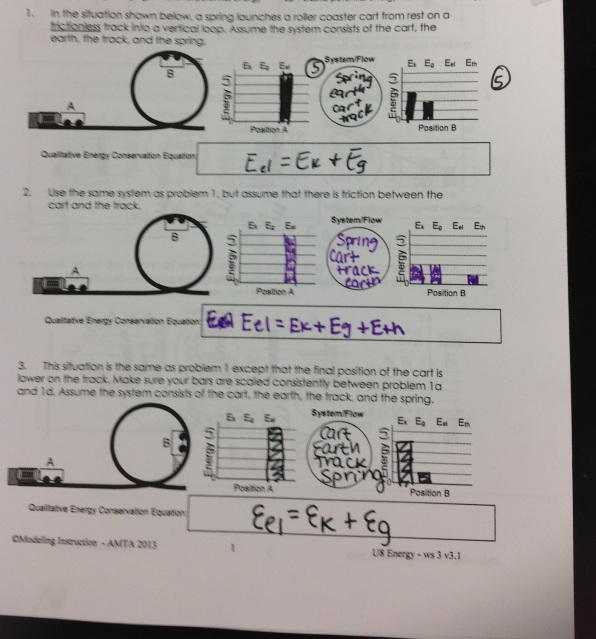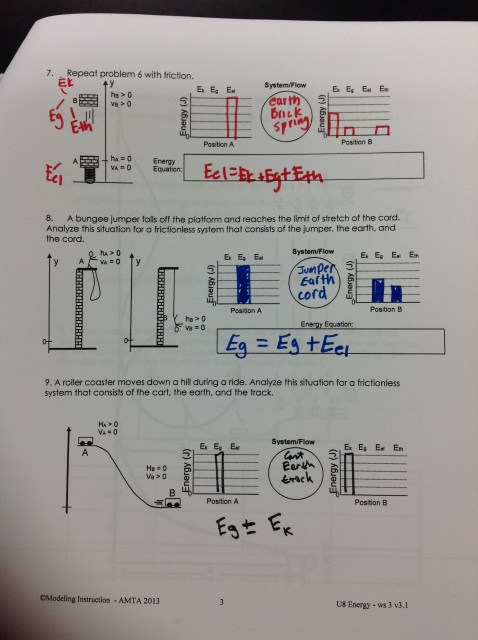# Qualitative Conservation of Energy

6 teachers like this lesson
Print Lesson

## Objective

Students will be able apply the law of conservation of energy to various situations qualitatively.

#### Big Idea

Students learn conservation of energy using qualitative bar graphs.

## Conservation of Energy Notes Recap

15 minutes

The goal of this lesson is to focus on the qualitative aspect of conservation of energy. Since we have looked at energy in general and students have taken notes on conservation of energy, I look at qualitative conservation of energy first so that students can better understand what it means for energy to be conserved (HS-PS3-2). When we jump right into quantitative problems, sometimes my students lose the conceptual aspect of conservation of energy which is equally important and makes completing the quantitative easier to accomplish. In this lesson, students are working on developing and using a graphical model for conservation of energy (SP2) as well as being able to create and equation that represents what type of energy is at one position versus another (SP5). By the end of this lesson, students are able to communicate what types of energy occur in any situation and how different parts of the situation are related through conservation of energy (SP8).

To start out the lesson, I give a mini-lesson on qualitative conservation of energy. I start by asking students to reflect on the video they watched for homework about conservation of energy and try to get at what they learned and write it on the board (as shown below). The most important thing that I want them to reflect on is that energy cannot be created or destroyed only transferred.Then I show them a situation of a cart being pushed up a frictionless hill by a spring. I use this example to introduce bar graphs. I start out by identifying the letters that symbolize each energy (Ek=kinetic energy, Eg=gravitational potential energy, Eel=elastic potential energy, Eth=themal energy). Then I ask students what type of energy exists in part A (elastic because of the spring). I emphasize that it doesn't matter how many bars I put for this one as long as the bars on the graph for part B are equal. (I use 5 cubes of height in all of my examples to be consistent.) Then I repeat the process with part B. I make sure to note that we don't know which type of energy (Ek or Eg) is more in this case but as long as the total number of boxes is equal to 5 our graph is correct. Finally, I end by creating the equation by looking at the types of energy from part A (initial) and setting it equal to the types of energy in part B (final).

After the first example, I do two examples using the same process of a child sliding down a slide. The difference between the two situations is that one slide is frictionless and the other is not. I find that it is good to do two examples with the same situation to show what difference friction makes on the graphs (as shown below).## Qualitative Energy Worksheet

25 minutes

After we review the conservation of energy notes from the video, I have students turn to the Qualitative Conservation of Energy WS in their packets. Students work with their table groups to complete this worksheet because when we go over the worksheet I ask each group to take one problem to put up on the answer key to share with the class. This worksheet really gets the students to think about what conservation of energy means by making sure there are the same number of bars total on each side as well as identifying which types of energy are in each part of the situation.

As students are working, I walk around to make sure students are on task and answer any general questions. The most common question is "Does it matter how many total blocks there are?" and I answer the initial and final should have the same total number of blocks but whatever number of blocks you choose is up to you as long as you are consistent. Additionally, I have questions about what the v and the h help us to understand and at times I stop the whole class to ask them that question to make sure that students understand v = velocity and that relates to kinetic energy and h = height and that relates to gravitational potential energy.

## Review Qualitative Energy Worksheet Answers

15 minutes

As groups are finishing up, I assign each group a problem. The problem that they are assigned must be copied to the worksheet used as an answer key. I have one member from each group come to my front table and write down the answer they got for their problem. I make sure that the worksheet is printed on three separate pages so that three students can be writing their answers down at a time. I go over the problems this way because it allow students to have a quick check of how they did as a group while still holding students accountable since they are putting their answers on the key.

When all of the answers are on the answer key, I share the answers page by page by using the document camera and projecting the answers on the front whiteboard. As I go through each page I ask students if they agree with all of the answers and if they do not to raise their hand and ask a question. After we go over any question for the page, I do a quick formative assessment of holding up the number of fingers that corresponds to the number of problems they got correct as a group. This helps me to know how each group did on the problems.

Here is the worksheet with the answers that my students put during one of my classes.## Stoplight Exit Slip

5 minutes

To end class, I use the stoplight exit slip to help students to process what they have learned. Each students takes a post-it note from the resource bin at the center of the table and gets to chose from the red, yellow or green light. They must complete the sentence starter on the light they choose.

The Red light: Today my learning stopped because...

The Yellow Light: Today I considered a question, new idea or perspective...

The Green Light: Today I learned __________ because...

When they complete their sentence, they stick the post-it note on whatever light they choose. The stoplight is at the front of the room whenever they finish writing their response to a light.

I do this to get an idea of where students are at in learning the material in a way that they can anonymously express exactly what they are learning or exactly what they are struggling with.